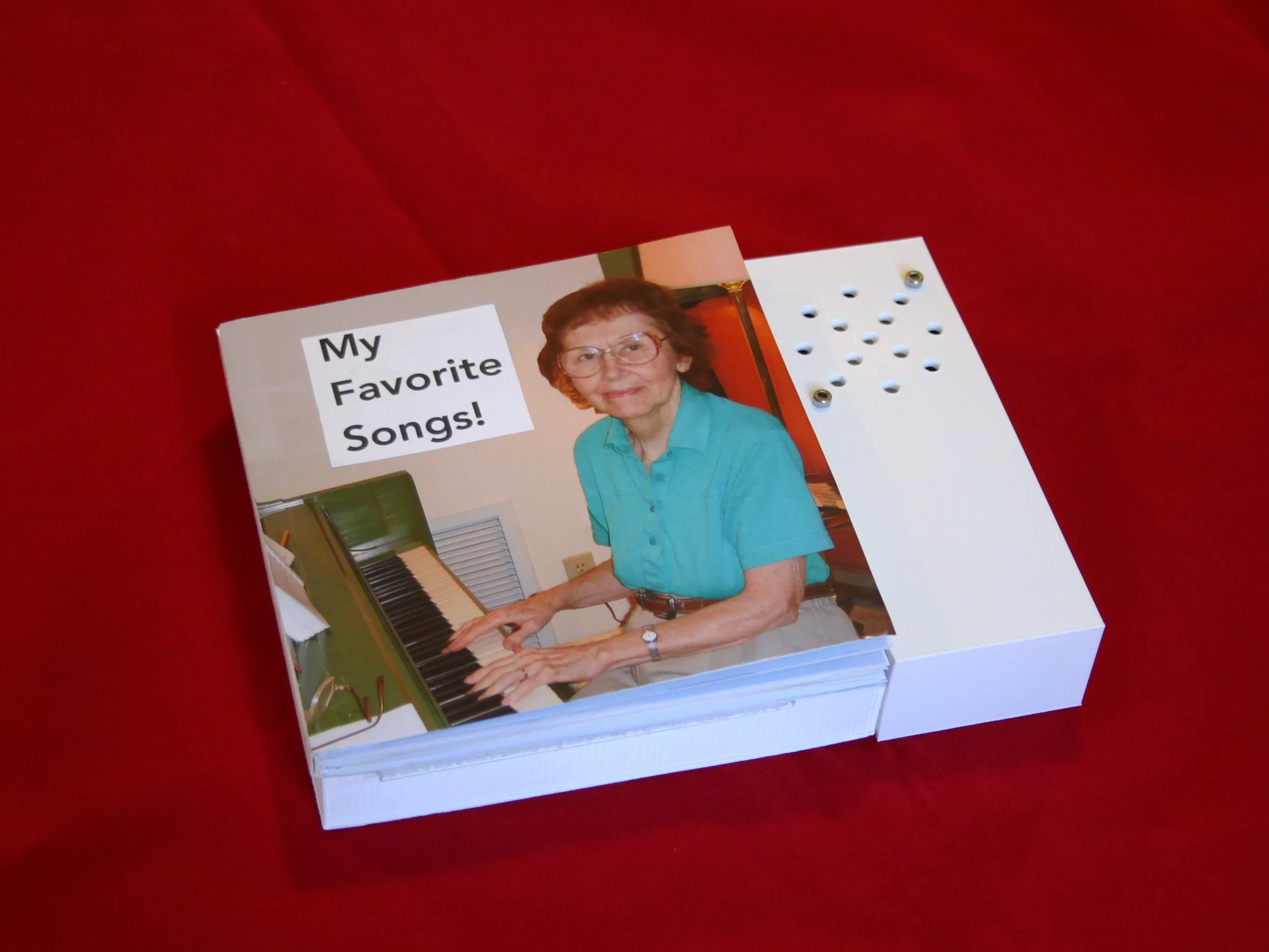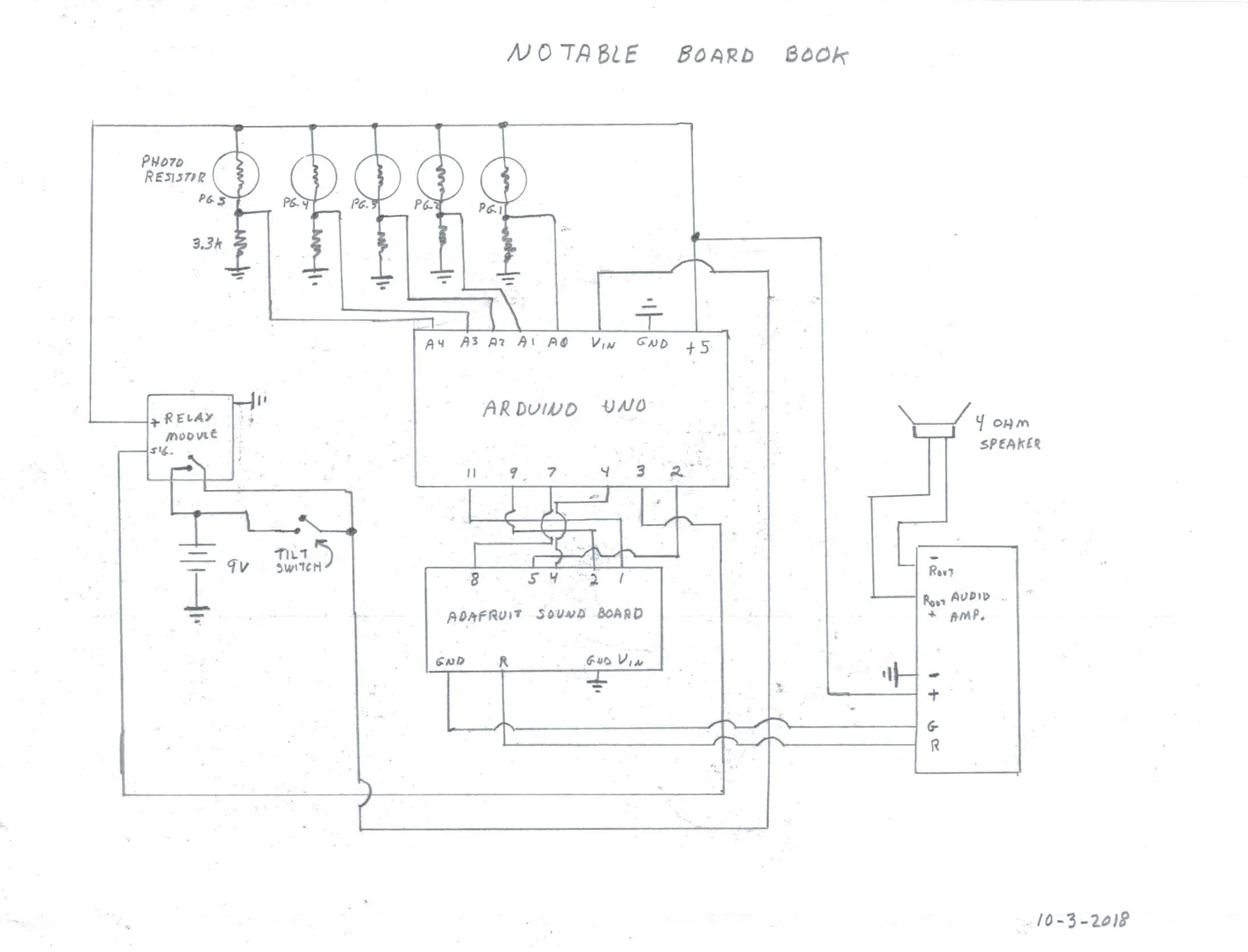Notable Board Books

How alert and happy Cecile (with Alzheimer's) became when she heard familiar songs! Lift the book, turn the page--the book does the rest.

AdvancedFull instructions provided20 hours2,741Things used in this project

Hardware componentsArduino UNO & Genuino UNO
×1

Hand tools and fabrication machines3D Printer (generic)

Schematics

SchematicCode

Notable Board Books Control (Arduino)

C/C++
int r=200;
int r1=200;
int r2=200;
int r3=200;
int r4=200;

int b=0;
int c=0;
int d=0;
int e=0;

int t1=0;//tally one
int t2=0;//tally two

int on=0;
int td=500;//time delay

void setup() {
pinMode (2,OUTPUT);
pinMode (4,OUTPUT);
pinMode (7,OUTPUT);
pinMode (9,OUTPUT);
pinMode (11,OUTPUT);

pinMode (3,OUTPUT);//set bypass relay
digitalWrite(3,HIGH);//hold system on

digitalWrite (2,HIGH);
digitalWrite(4,HIGH);
digitalWrite(7,HIGH);
digitalWrite(9,HIGH);
digitalWrite(11,HIGH);

delay(1000);//wait while book is being picked up

}

void loop() {

if (r4>600) {
a=1;
}
if (r3>600) {
b=2;
}
if (r2>600) {
c=4;
}
if (r1>600) {
d=8;
}
if (r>600) {
e=16;
}
t1= a+b+c+d+e;

if (r4>600) {
a=1;
}
if (r3>600) {
b=2;
}
if (r2>600) {
c=4;
}
if (r1>600) {
d=8;
}
if (r>600) {
e=16;
}
t2= a+b+c+d+e;

if (t1==t2) {//valid read twice in a row

if (a==1) {
digitalWrite(2,LOW);
on=1;
digitalWrite(4,HIGH);
digitalWrite(7,HIGH);
digitalWrite(9,HIGH);
digitalWrite(11,HIGH);

digitalWrite(3,HIGH);
delay(150);//keep pin low for sound fx board

}
if ((b==2) && (on==0)){
digitalWrite(4,LOW);

digitalWrite(2,HIGH);
digitalWrite(7,HIGH);
digitalWrite(9,HIGH);
digitalWrite(11,HIGH);

digitalWrite(3,HIGH);
on=1;
delay(150);

}
if ((c==4) && (on==0)) {
digitalWrite(7,LOW);
digitalWrite(4,HIGH);
digitalWrite(2,HIGH);
digitalWrite(9,HIGH);
digitalWrite(11,HIGH);

digitalWrite(3,HIGH);
on=1;
delay(150);

}

if ((d==8) && (on==0)){

digitalWrite(9,LOW);
digitalWrite(4,HIGH);
digitalWrite(7,HIGH);
digitalWrite(2,HIGH);
digitalWrite(11,HIGH);

digitalWrite(3,HIGH);
on=1;
delay(150);

}
if ((e==16) && (on==0)) {
digitalWrite(11,LOW);
digitalWrite(4,HIGH);
digitalWrite(7,HIGH);
digitalWrite(9,HIGH);
digitalWrite(2,HIGH);

digitalWrite(3,HIGH);
delay(150);
}

if(t2==0) {
digitalWrite(11,HIGH);
digitalWrite(4,HIGH);
digitalWrite(7,HIGH);
digitalWrite(9,HIGH);
digitalWrite(1,HIGH);
digitalWrite(3,LOW);//turn off bypass relay
}}

on=0;
a=0;
b=0;
c=0;
d=0;
e=0;

}

Credits

Mike Rigsby

6 projects • 23 followers
I am an author and a maker. Current projects include Santa's Shop and Little Friend (ultracapacitor powered robot) on hackaday.io.
Thanks to Annelle Rigsby and Tia Rigsby.